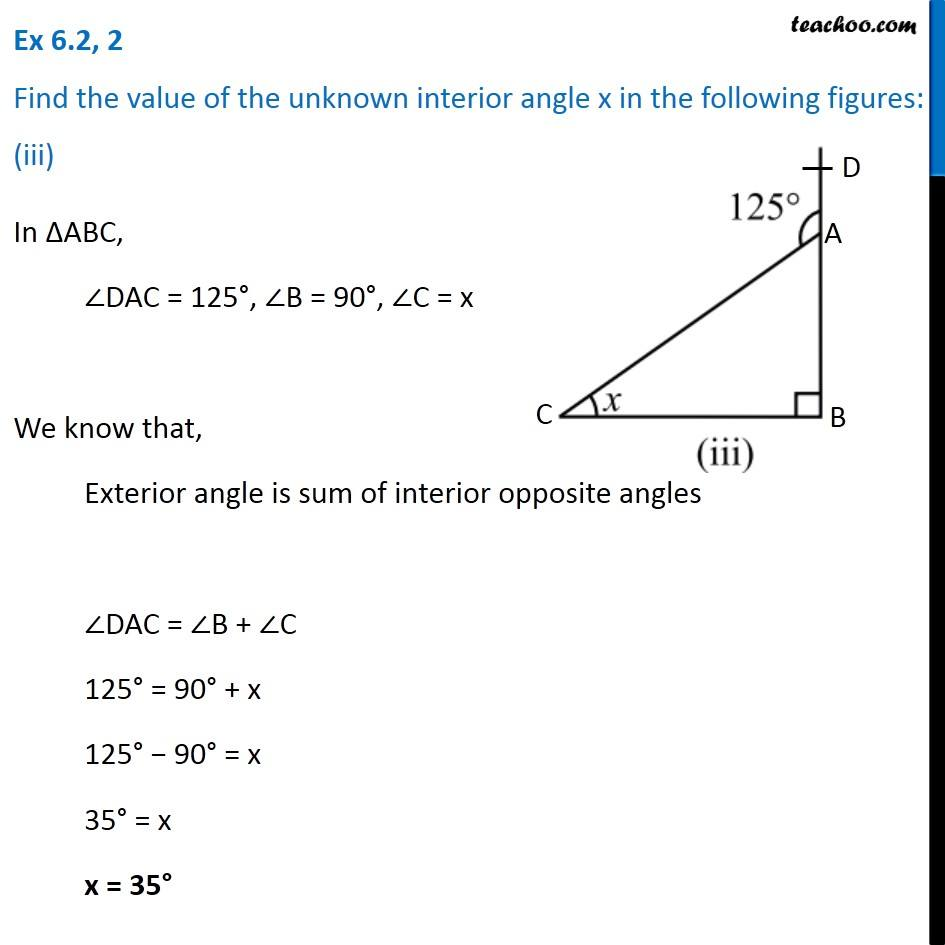Ex 6.2

Chapter 6 Class 7 Triangle and its Properties
Serial order wiseLearn in your speed, with individual attention - Teachoo Maths 1-on-1 Class

### Transcript

Ex 6.2, 2 Find the value of the unknown interior angle x in the following figures: (iii)In ∆ABC, ∠DAC = 125°, ∠B = 90°, ∠C = x We know that, Exterior angle is sum of interior opposite angles ∠DAC = ∠B + ∠C 125° = 90° + x 125° − 90° = x 35° = x x = 35°